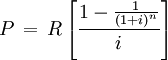SEARCH HOMEMath Central Quandaries & QueriesQuestion from Jules, a student: R 20 000 is deposited in the bank,at the end of each year R 5 000 is withdrawn from it, the interest is 13 percent compounded monthly. Calculate how many years that person will be able to use his/her money ?Hi Jules.

Use the formula for annuities.where:
P is the principal (R 20 000)
R is the withdrawal (R 5 000)
i is the interest rate (0.13)
and n is the number of withdrawals. That's what you need to find.

You'll find it by re-arranging the equation and using logarithms, or you could estimate it by graphing an exponential function and finding the intersection with a line.

Write back if you need more help.

Cheers,
Stephen La Rocque.Math Central is supported by the University of Regina and The Pacific Institute for the Mathematical Sciences.